## Graph of graphs

The pictures1 in this page represent the space of trivalent graphs with various number of vertices. This space has a natural geometry, namely, two graphs are close if they differ by a few local mutations.

More precisely, we can give the space of graphs the structure of a graph itself. Let GG(n) be the graph whose vertices are trivalent graph with n=2k vertices and two vertices are connected by an edge if the associated graphs differ by a single Whitehead move.There are two Whitehead moves possible for every edge.
We are interested in the geometry of this graph. The graph GG(n) is always connected. The number of its vertices is of order n(n/2) (see for example Bollobas) and its diameter is comparable to ( n log n ) (see Rafi-Tao). Here is depiction of GG(6):The graph GG(6).
The graph GG(n) is natrually stratified by girth.(Recall that girth of a graph is the length of its shortest loop.) Let GG(n,g) be the subgraph of GG(n) consisting all graphs that have girth at least g. Here, we examine if these subgraphs are themselves connected.

#### Simple graphs

A graph is called simple if it has girth at least 3 (no loops or double edges). It was shown by Coco Zhang2 that the subgraphs GG_simple(n) and GG_not_simple(n) are both connected. Hence to simplify our discussion, we concentrate on simple graphs only. We refer to GG_simple(n) = G(n) and to the subgraph of G(n) consisting of graphs of girth at least g by G(n,g).

Here are a few pictures of the graph of simple graphs. The vertices are colored by the girth of the associated graph as follows:
girth 3: blue;    girth 4: green;    girth 5: cyan;    girth 6: magenta;    girth 7: yellow;    girth 8: red;G(6)G(8)G(10)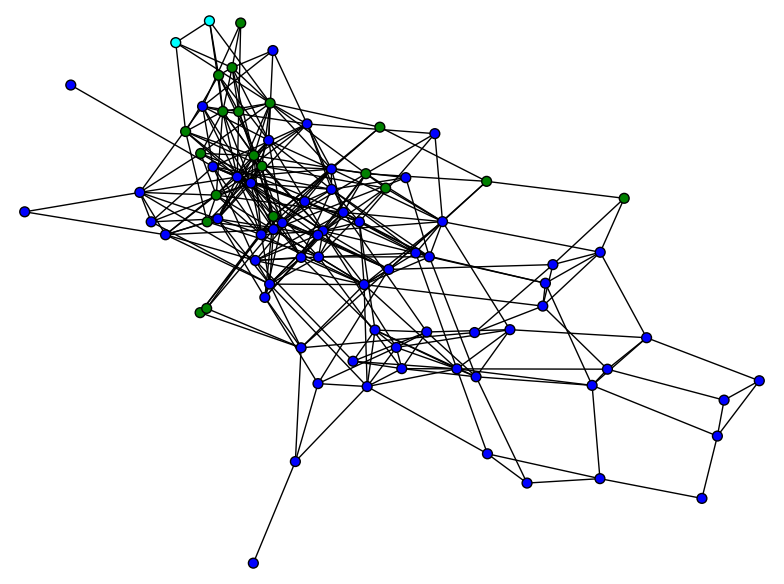G(12)
At first each strada seems to be connected. On the right, we have the picture of the slice with the same color coding as before.

We define G(n,k) to be the subgraph of G(n) induced by the vertices(graphs) with girth ≥ k.G(14)G(14,4)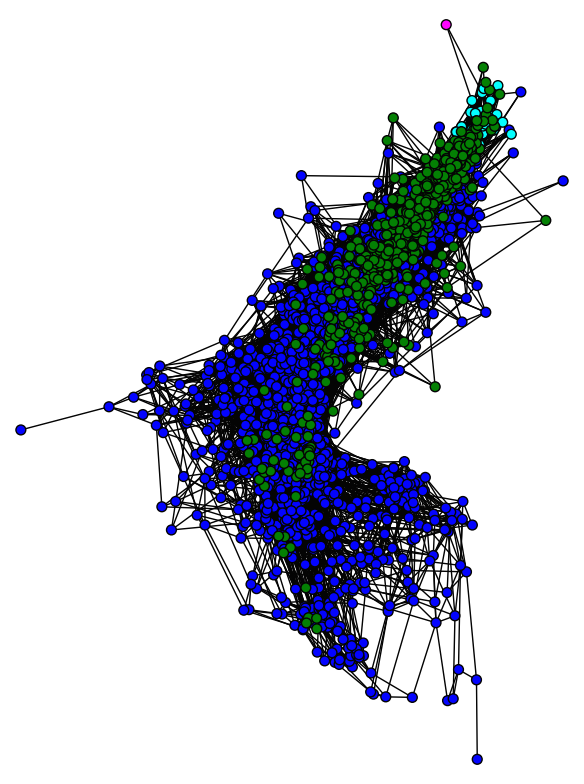G(16)G(16,5)
But for larger values of n this is not true. For n = 18 and 20, the graphs of girth 3 and 4 are omitted to make the picture less crowded.G(18,5)G(18,6)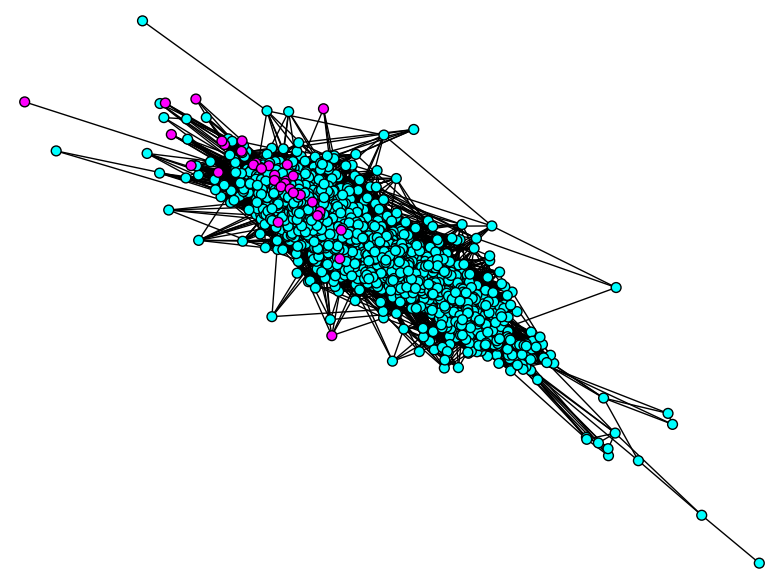G(20,5)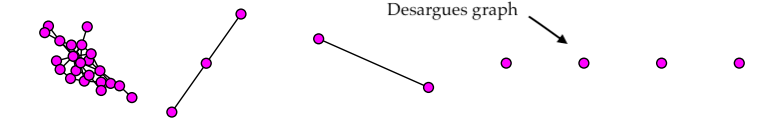G(20,6)
For n=22, there are 90553 graphs of girth 5. Hence, we just depict the girth ≥ 6 subgraph, which is again disconnected.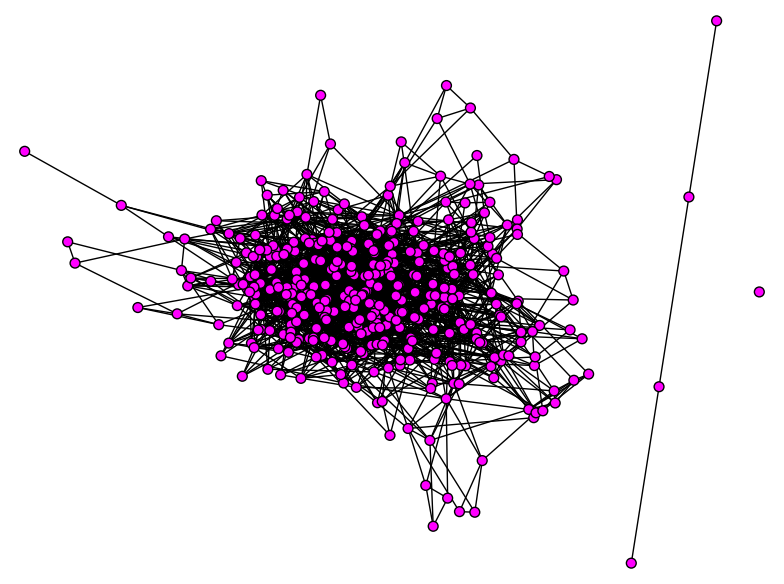G(22,6)

It seems like the "end" of G(n) is somewhat tree like. It is a question of McMullen whether this family of graphs is an expander family.

#### c-covered graphs and connectivity of G(n,k) 3

To study the general rules of connectivity in G(n), we define the folowing term:

Definition:
A graph is c-covered by short cycles (or "c-covered" for short) if every path of length c is contained in a cycle of length k, where k is the girth of graph.

Example 1:
The Desargues graph (n=20, girth=6) is 3-covered by short cycles.

The following pictures are two representations of Desargues graphs.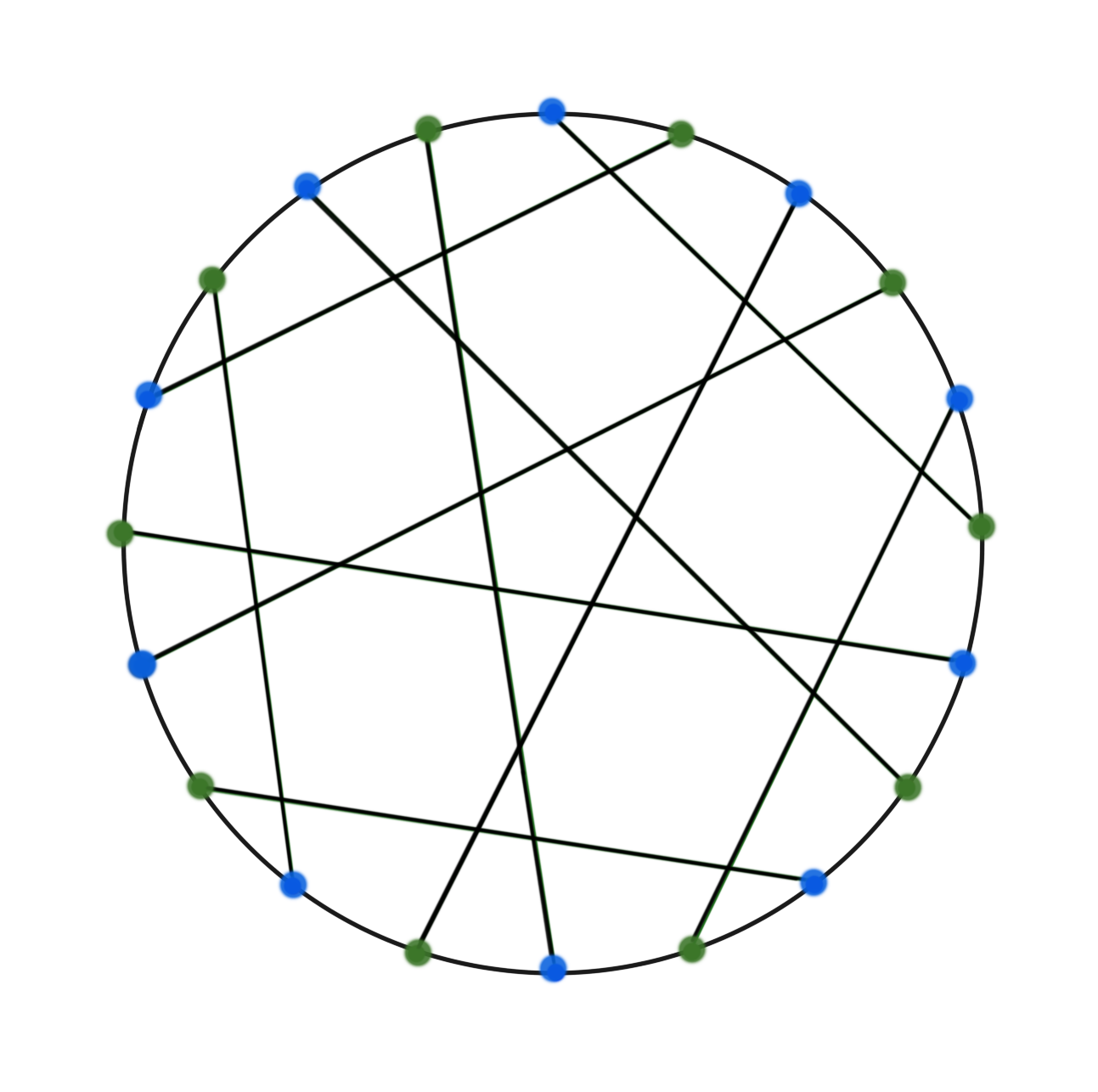Desargues graph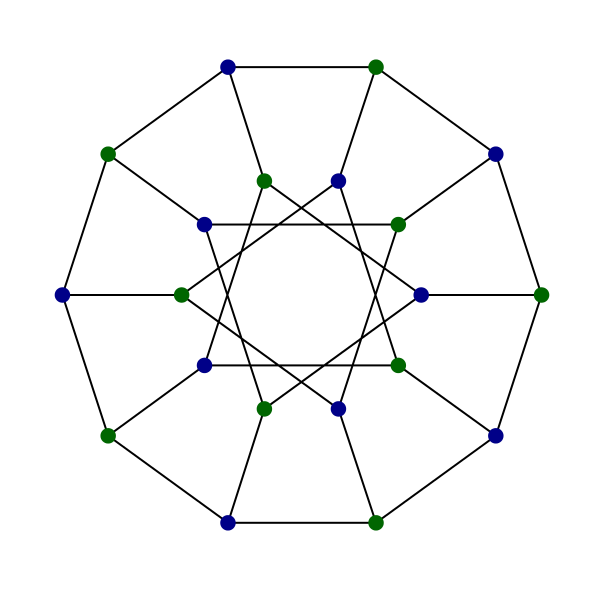Desargues graph
The Desargues graph is distance-transitive, which means given any two vertices v and w at any distance i, and any other two vertices x and y at the same distance, there is an automorphism of the graph that carries v to x and w to y.

Therefore, if we pick a path P of length 3 in the Desargues graph, there exists an automorphism that maps the path to a-b-c-d depicted below. We see that there is a cycle of length 6 (a-b-c-d-e-f-a) containing the path a-b-c-d. Now, the inverse automorphism send the cycle a-b-c-d-e-f-a to a cycle that contain P. By a systole we mean a cycle that has a length equal to the girth of the graph. Thus any path of length 3 is contained in a systole and hence the Desargues graph is 3-covered.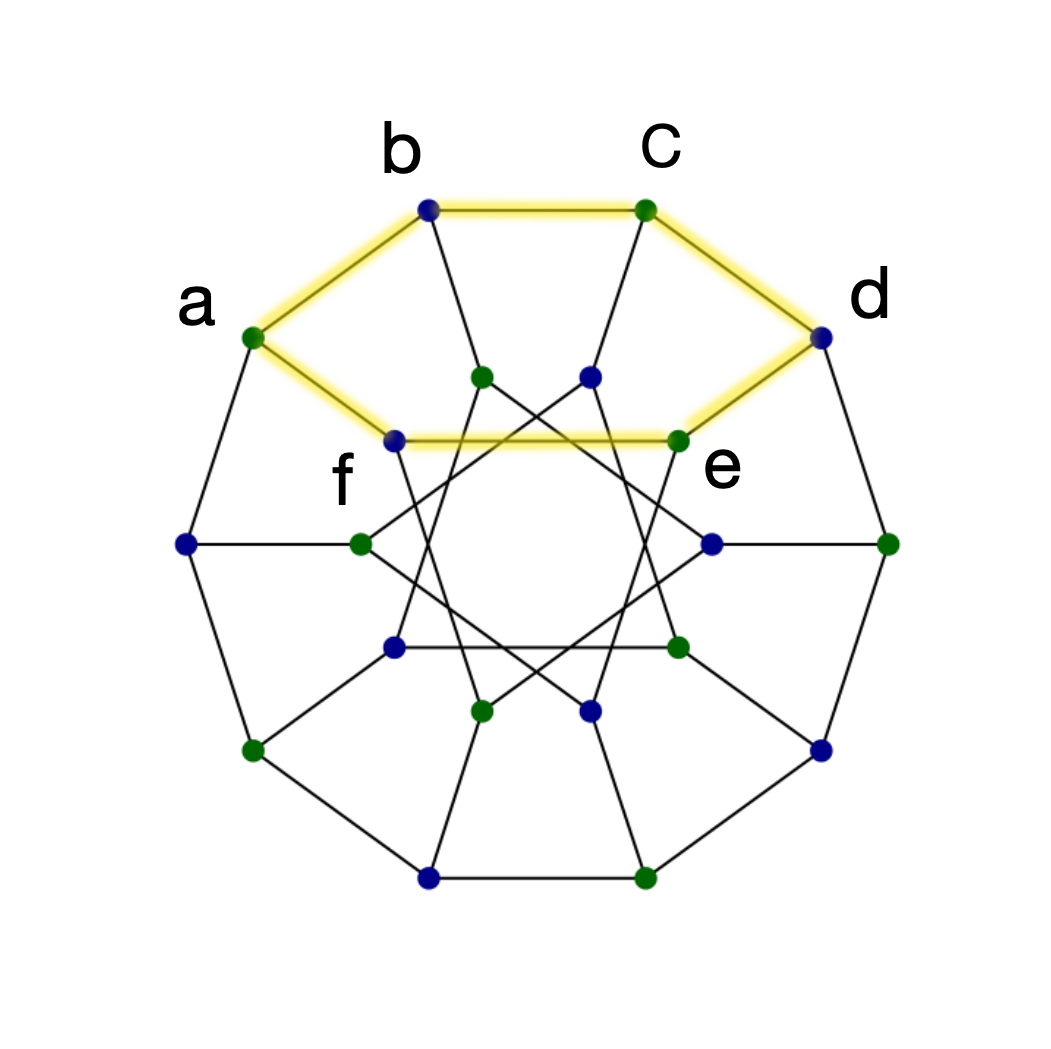systole a-b-c-d-e-f-a
Similar argument shows that if a trivalent distance-transitive graph has girth ≥ 6, then it is 3-covered by short cycles. There are 12 finite trivalent distance-transitive graphs4, 7 of which have girth ≥ 6.

 Graph name Number of Vertices Girth Heawood graph 14 6 Pappus graph 18 6 Desargues graph 20 6 Coxeter graph 28 7 Tutte-Coxeter graph 30 8 Foster graph 90 10 Biggs-Smith graph 102 9

These special graphs are indicated in the plots of G(n) and G(n,k).
Example 2:
Generalized Petersen graph GP(3m+3, m) is 3-covered by short cycles, m ∈ N, m ≥5.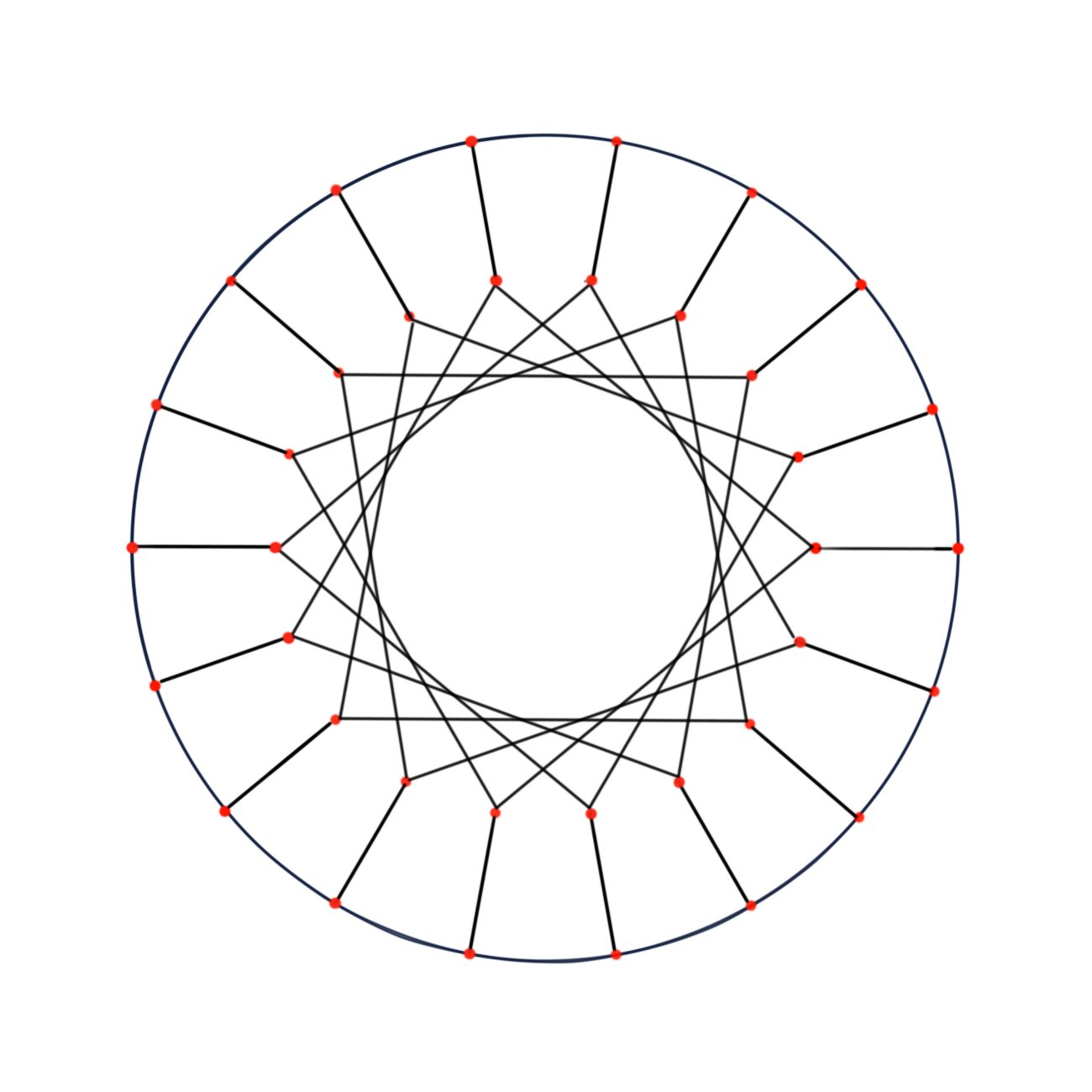GP(18, 5)

Consider the case when m=5, i.e. GP(18, 5). The length of the shortest cycle is 8, thus girth = 8. And there are two types of systoles in it.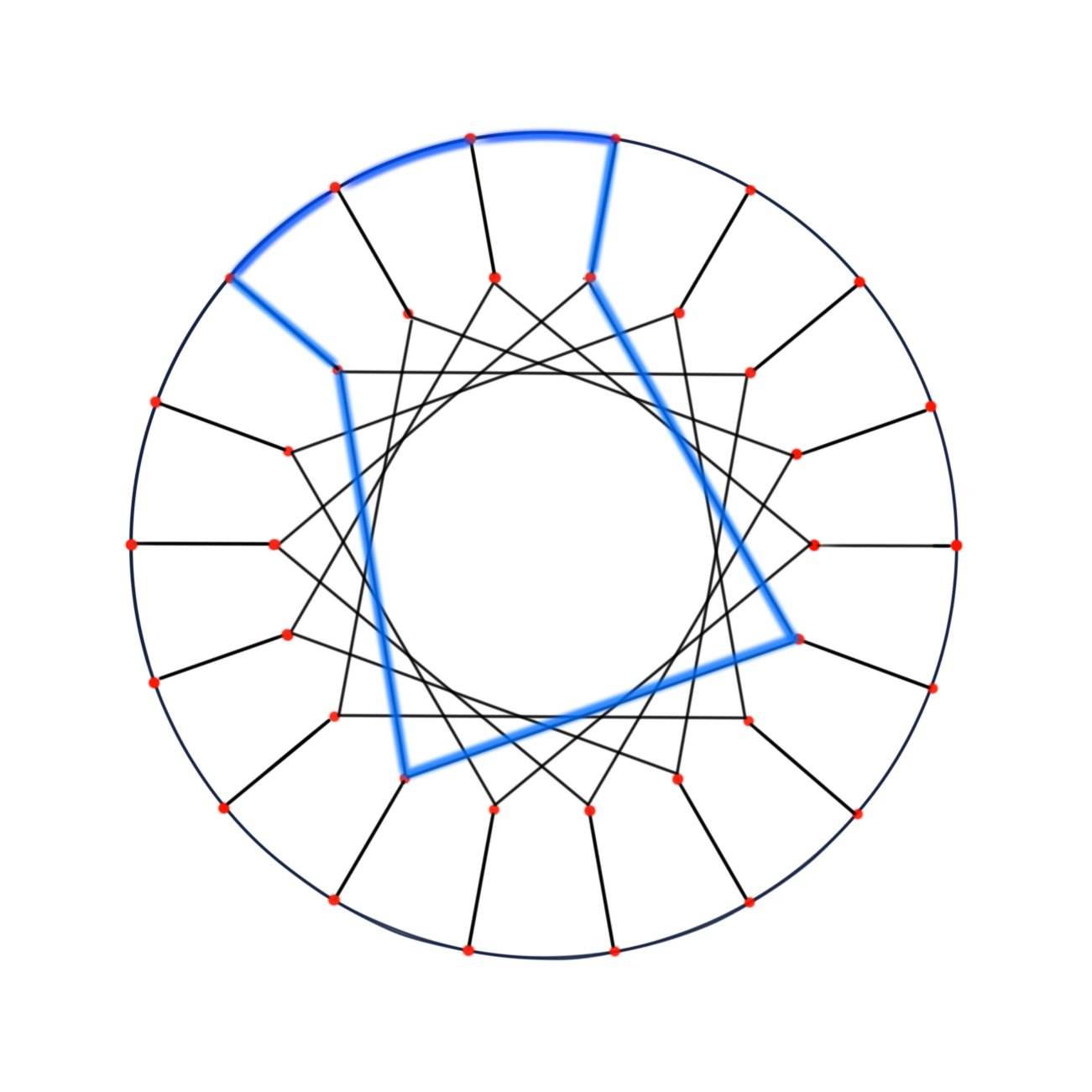Type IType II
To show GP(18, 5) is 3-covered, we classify edges into a, b, c as following:GP(18, 5)
Any path of length 3 can be represented as one of the following:
a-a-a, a-a-b (b-a-a), a-b-c (c-b-a), b-c-c (c-c-b), b-c-b.

a-a-a, a-a-b(b-a-a), b-c-c is contained in Type I systole;
b-c-b is contained in Type II systole;
a-b-c(c-b-a) is contained in Type I or Type II systole, depending on orientation of c.

From the argument above, any path of length 3 in GP(18, 5) is contained in a cycle of length 8. Therefore, GP(18,5) is
3-covered by short cycles. This is also true for other values of m, m ∈ N, m ≥5.GP(3m+3, m)
The property of being "3-covered by short cycles" is important to the connectivity of G(n,k).

Theorem:
In G(n), every 3-covered graph with girth k only connects to graphs with girth < k.
In other words, any Whithead move on 3-covered graph will decrease the girth.

Proof:
Let g be a 3-covered graph with girth k in G(n). Then every path of length 3 is contained in a cycle of length k.Suppose one Whitehead move is operated on an arbitrary edge e in g. Before the operation, path e1-e-e3 and e1-e-e4 are both contained in cycles of lengths k. After the operation, either e1-e-e3 will be shortened to e1-e3 (case1), or e1-e-e4 will be shortened to e1-e4 (case2). In either case, the length of the systole containing e1-e-e3 or e1-e-e4 will decrease by 1. ∎

Now recall that G(n,k) is a subgraph of G(n) induced by the vertices (graphs) with girth ≥ k. If G(n,k) contains only vertices (graphs) of girth k and one of these graphs is 3-covered by short cycles, then the graph is contained in a disconnected component of G(n,k).

For convenience, if the subgraph G(n,k) is disconnected and the vertices (graphs) have j different levels of girths, we call this property of G(n,k) by j-level disconnectivity. G(18,6), G(20,6) and G(22,6) above are 1-level disconnected.

Here are two examples of 2-level disconnectivity.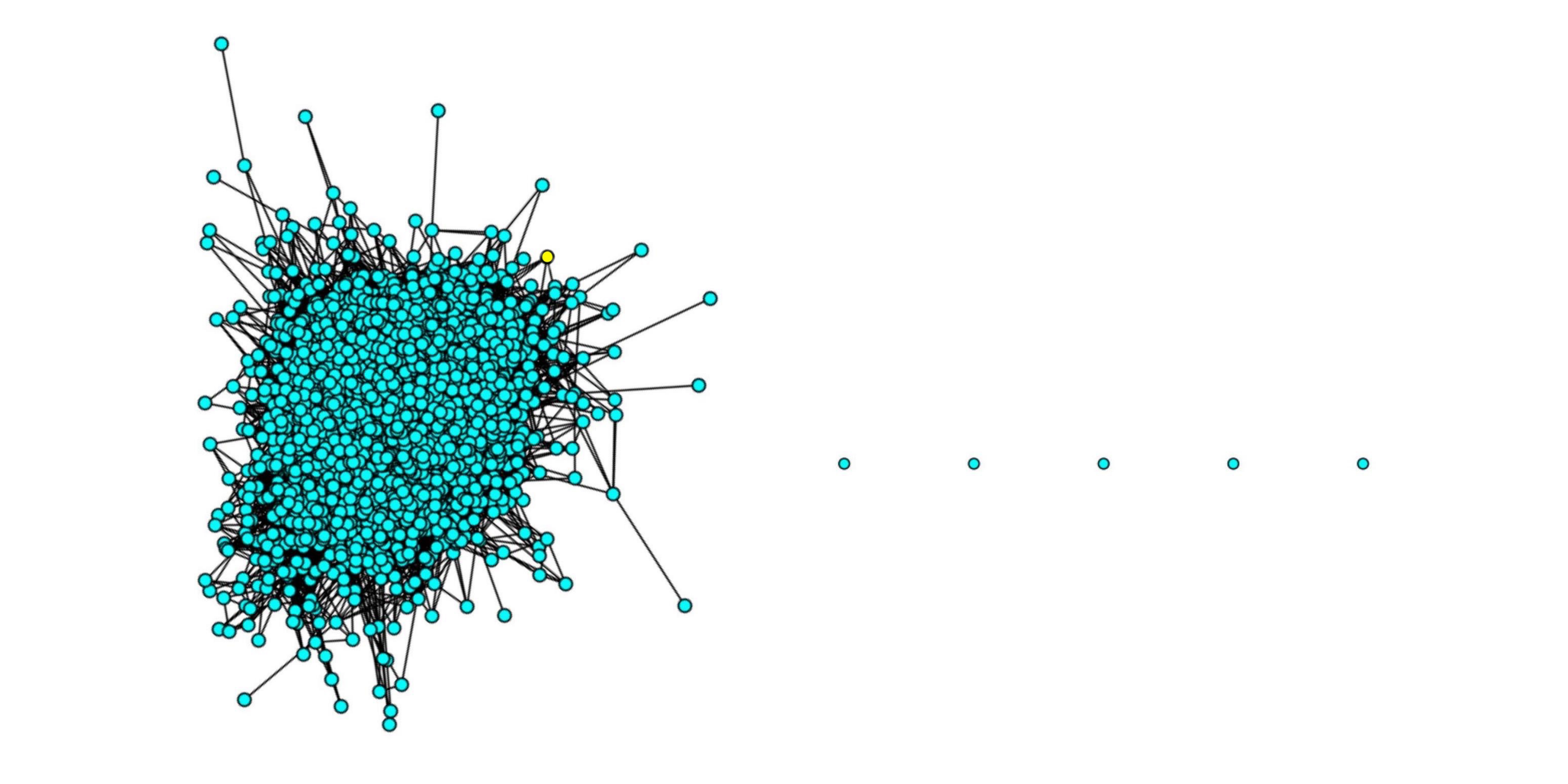G(24,6) contains vertices of girth 6 (cyan) and 7 (yellow)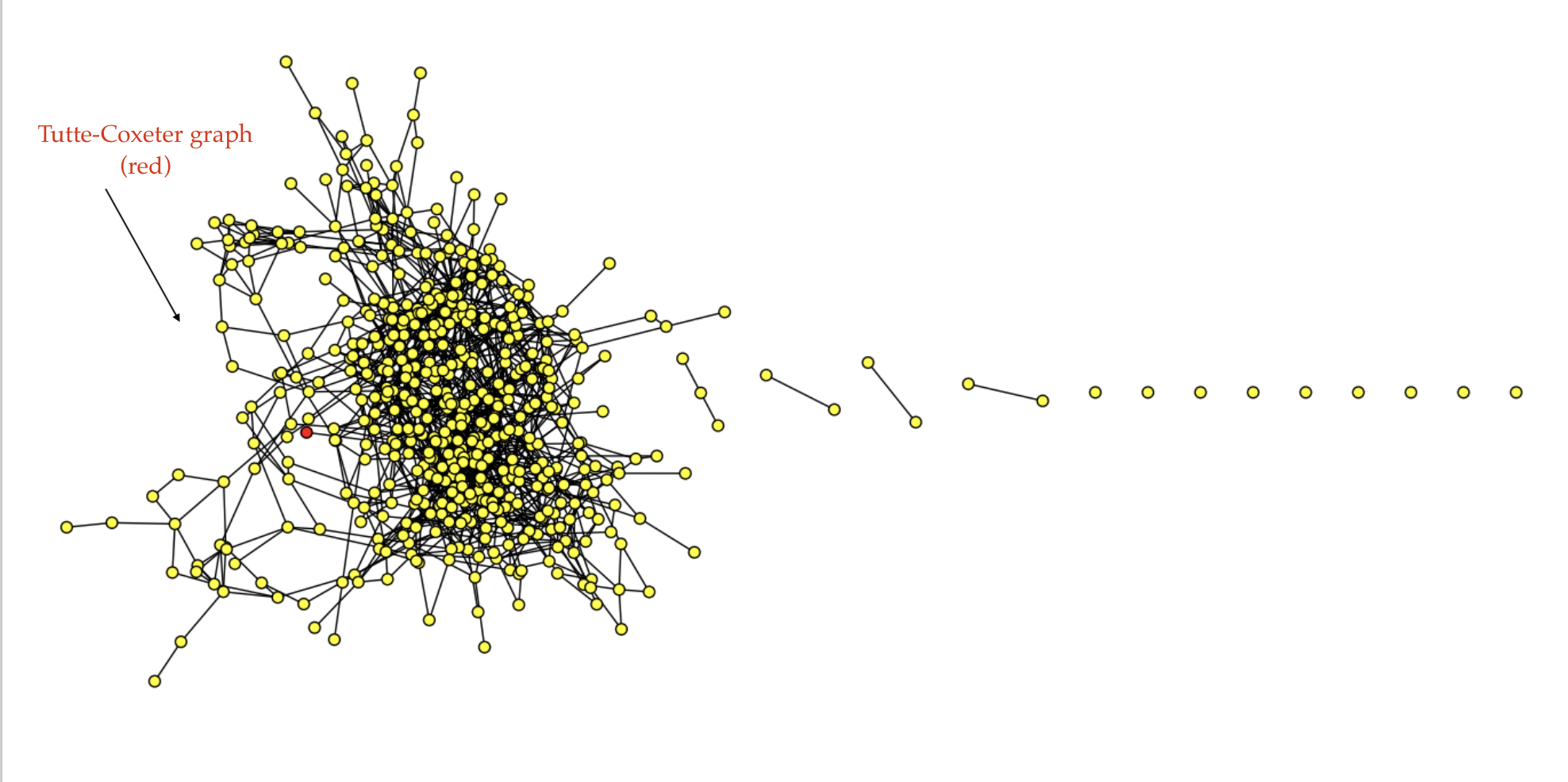G(30,7) contains vertices of girth 7 (yellow) and 8 (red)
It seems that when n and k are large enough, the value of j for j-level disconnectivity is greater. However, the problem becomes rather computationally difficult for larger n and k.

It is a question of Ci whether the property of c-covered of the vertices (graphs) in G(n,k) is related to the level of disconnectivity of G(n,k). Does a greater value of c imply more disconnectivity levels?

Here is a table of the number of trivalent graphs of a given girth. 5

 Vertices girth = 3 girth = 4 girth = 5 girth = 6 girth = 7 girth=8 4 1 6 1 1 8 3 2 10 13 5 1 12 63 20 2 14 399 101 8 1 16 3,268 743 48 1 18 33,496 7,350 450 5 20 412,943 91,763 5,751 32 22 5,883,727 1,344,782 90,553 385 24 94,159,721 22,160,335 1,612,905 7,573 1 26 1,661,723,296 401,278,984 31,297,357 181,224 3 28 31,954,666,517 7,885,687,604 652,159,389 4,624,480 21 30 663,988,090,257 166,870,266,608 14,499,780,660 122,089,998 545 1 32 14,814,445,040,728 3,781,101,495,300 342,646,718,608 3,328,899,586 30,368 0 34 ? ? ? 93,988,909,755 1782839 1 36 ? ? ? 2,754,127,526,293 95,079,080 3

#### Footnotes

1. 1- These pictures have been produce in collaboration with Roi Docampo, Jing Tao and Ci Zhang using Sage.
2. 2- In 2013, Coco Zhang was a high school junior at Branksome Hall. This work was done as part of the Math Mentorship Program at the University of Toronto during the winter of 2013.
3. 3- This section is written by Ci Zhang as part of her Work Study during the Summer and Fall 2018.
4. 4- Biggs, N. L.; Smith, D. H. (1971), "On trivalent graphs", Bulletin of the London Mathematical Society, 3 (2): 155–158, see here. MR 0286693.
5. 5- Source: http://oeis.org/A198303. See also here